## Oscillators

Dec 2, 2009

### Introduction:

This article demonstrates examples of Wien-bridge, LC-tuned and crystal oscillators covering the range of kHz to 100 MHz.

### Wien-bridge op amp oscillators:

The classic super low distortion Wien-bridge oscillator with 1869 lamp stabilization and LM4562 50 MHz, 20 V/us opamp. Useful to about 500 kHz.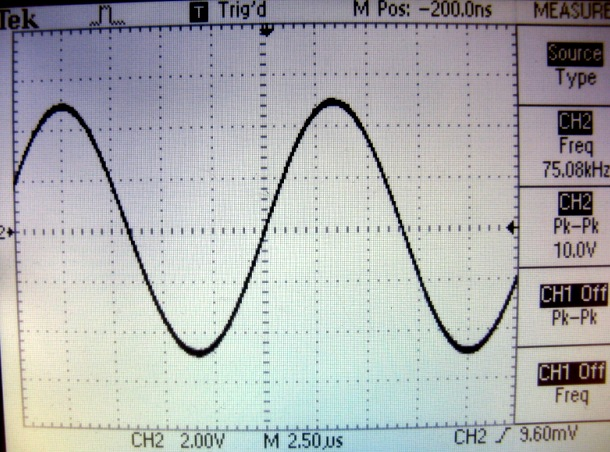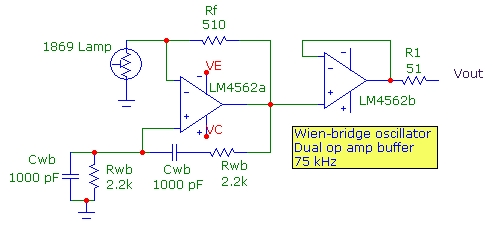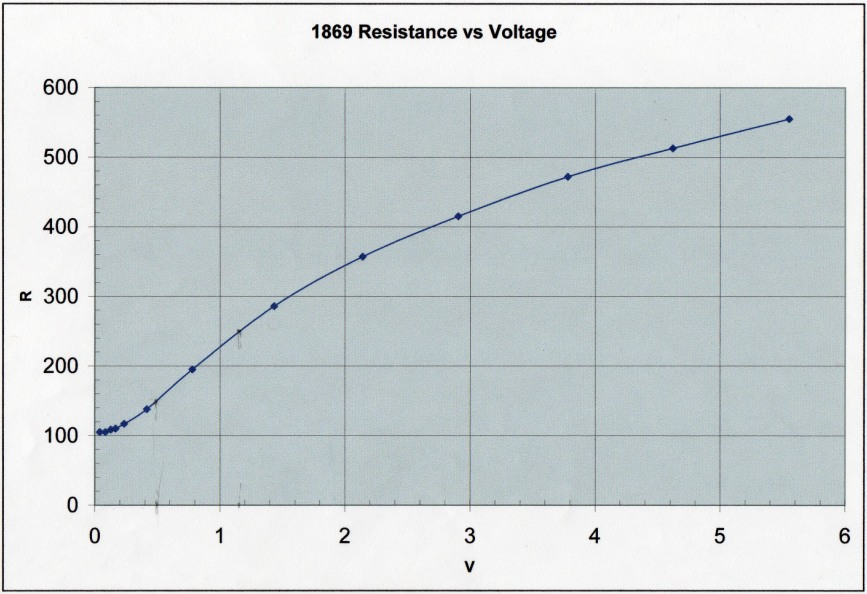The incandescent lamp resistance as a function of DC (or RMS AC) voltage shown above can be used to determine the peak output oscillation Vpo. The Wien-bridge phase characteristic and Barkhausen criterion requires that the feedback resistance ratio Rf/Rlamp be exactly 2 where Rlamp is determined by the average heating effect of the oscillation voltage across it. (This assumes that the incandescent lamp device behaves as a pure resistance at the frequency of oscillation.) The PEAK voltage across the lamp at oscillation is therefore Vpo*Rlamp/(Rf + Rlamp) = Vpo/3. The corresponding RMS lamp voltage Vlamp equivalent to the DC voltage shown in the graph above is therefore Vpo/(3*Sqrt(2)) or:
Vpo = 3Sqrt(2)*Vlamp
For example, the circuit above uses Rf=510 ohm. The lamp resistance at oscillation must be exactly half this or 255 ohm. From the graph, the lamp voltage for this resistance value is Vlamp = 1.15VDC so the peak output oscillation voltage is 4.88V, in agreement with the oscilloscope trace above. With Rf = 300 ohm, Rlamp = 150 ohm at Vlamp = 0.5VDC and Vop = 2.1V. With this lamp, the minimum usable value of Rf is about 220 ohm, Rlamp = 110 ohm, Vlamp ~ 0.2V and Vop ~ 0.85V.

Wien-bridge oscillator with diode limiter stabilization. More distortion than lamp stabilization and must be adjusted to just oscillate:To minimize diode-limiter distortion, the feedback loop is adjusted using the trimmer Rib so that the circuit just starts to oscillate corresponding to Rf/(Ria + Rib) being slightly greater than 3 (since the positive feedback fraction for the Wien bridge is 1/3). For 1N4148 Si diodes, the forward diode voltage just at start of oscillation is ~ 0.45 V. The ratio of output resistors R5, R6 along with Vcc determines the output oscillation amplitude. Simple node analysis shows that:
Vout(peak) = 3[(Vcc + Vdiode)R5/R6 + Vdiode]/(2 - R5/R6)
with Vdiode 0.4 - 0.5V.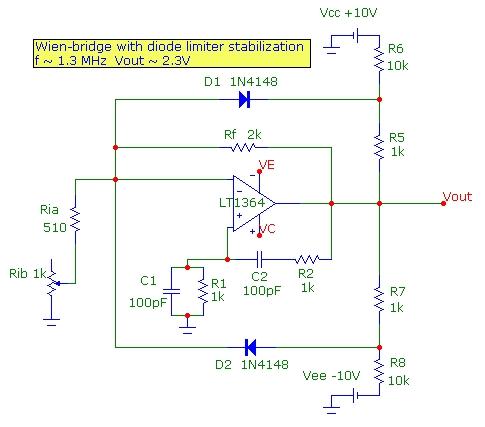### Colpitts and Clapp Transistor Oscillators:

A 24 MHz Colpitts oscillator in the common emitter configuration with high-speed 100 MHz LM6171 op-amp output buffer.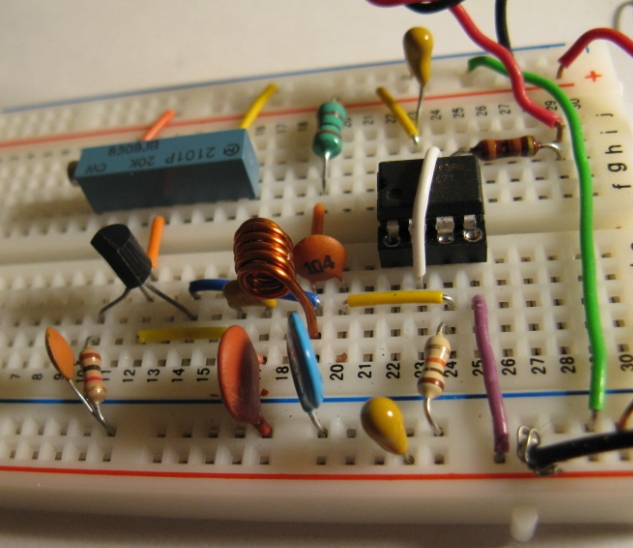A 71 MHz Clapp oscillator in the common-base configuration. The CB configuration removes the Miller capacitance loading and bandwidth reduction of the CE configuration enabling higher oscillation frequency. Useful up to 100 MHz: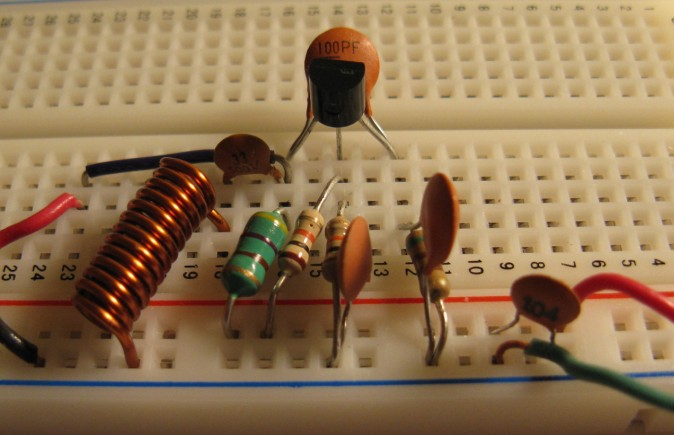### Crystal op amp oscillator:

This circuit uses an LM6181 current-feedback op amp with a 10 MHz crystal and diode stabilization against the input op amp circuit. Provides buffered high output drive of ~100 mA. See RF oscillator uses current-feedback op amp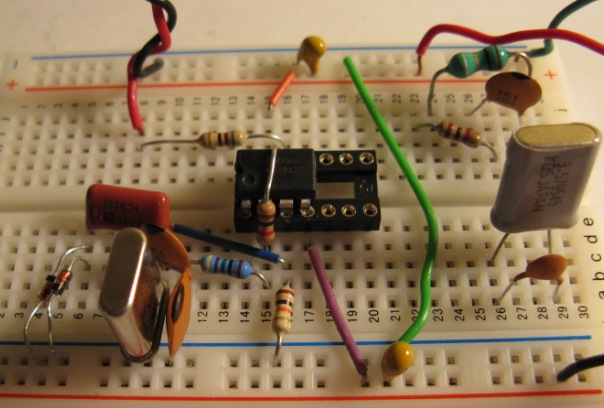## References

• Sine Wave Generation Techniques, National Semiconductor Application Note 263
• Electronic Principles, A. Malvino, 3rd Edn. McGraw Hill, 1984
• Microelectronic Circuits, A. Sedra and K. Smith, 2nd Edn. Holt, Rinehart and Winston, 1987 p.724
• IC OP-AMP Cookbook, Walter Jung, 2nd Edn. 1980 SAMS.